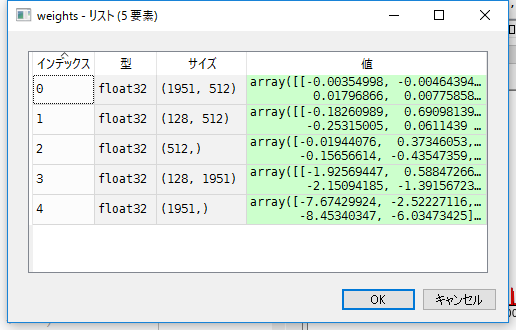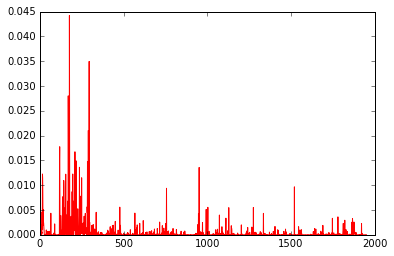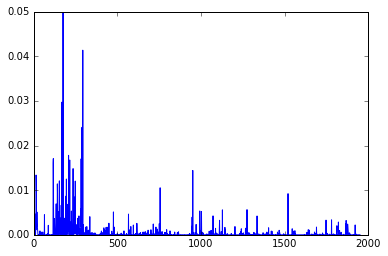# Overview

I used Keras and Tensorflow to calculate the weights of the neural network, but it's surprisingly difficult to know how to use it in real applications (iPhone apps, Android apps, Javascript, etc.).

If it is a simple neural network, it is simple, but since it was necessary to use the weights learned in LSTM this time, I deciphered the contents of Predict of LSTM of Keras.

The learned weights can be retrieved with `model.get_weights ()`, but no information about this one comes out even if I google it.

After all, as a result of writing the code and trying it randomly, the weights fetched by `model.get_weights ()` are First (index 0): LSTM input layer weights for inputs, input gate weights, output gate weights, forgetting gate weights Second (index 1): Hidden layer input weights, input gate weights, output gate weights, forgetting gate weights Third (index 2): Bias for input layer and hidden layer Fourth (index 3): Weight for output layer input (weight for hidden layer output) Fifth (index 4): Bias for output layer (bias for hidden layer output) I found out.

To figure this out, I wrote the code that (will) behaves like model.predict () at the end.

I hope the official page of Keras has something like this. .. .. ..

# Output of get_weigts ()

If `weights = model.get_weights ()`, the following weights are stored.The number 1951 is the number of nodes in the input layer, so there is no problem. Index 3 128 also has the number of hidden layer nodes set to 128, so it can be seen as a weight for the output of the hidden layer. The problem was 512, which was the most confusing.

I suddenly came up with the expectation that four types of "weights for inputs, weights for input gates, weights for output gates, and weights for forgetting gates" are stored. However, looking at the official Keras page, there is a statement that LSTM uses (long-term memory unit-Hochreiter 1997.). Speaking of 1997, the oblivion gate wasn't weighted. .. .. ?? It seems that the oblivion gate was created in 1999. .. .. .. That's what it says here too ...? http://kivantium.hateblo.jp/entry/2016/01/31/222050

I was confused by that, but I thought that if I wrote the code in solid, these would be clarified, so I decided to write this code that behaves the same as model.predict.

# Code to unravel model.predict ()

As a premise, the model of LSTM used for decoding this time is as follows. Sample code to generate sentences using LSTM.

``````from __future__ import print_function
from keras.models import Sequential
from keras.layers import Dense, Activation
from keras.layers import LSTM
from keras.optimizers import RMSprop
from keras.utils.data_utils import get_file
import numpy as np
import random
import sys
from keras.models import model_from_json
import copy
import matplotlib.pyplot as plt
import math

#path = get_file('nietzsche.txt', origin='https://s3.amazonaws.com/text-datasets/nietzsche.txt')
print('corpus length:', len(text))

chars = sorted(list(set(text)))
print('total chars:', len(chars))
char_indices = dict((c, i) for i, c in enumerate(chars))
indices_char = dict((i, c) for i, c in enumerate(chars))

# cut the text in semi-redundant sequences of maxlen characters
#maxlen = 40
maxlen = 3
step = 2
sentences = []
next_chars = []
for i in range(0, len(text) - maxlen, step):
sentences.append(text[i: i + maxlen])
next_chars.append(text[i + maxlen])
print('nb sequences:', len(sentences))

print('Vectorization...')
X = np.zeros((len(sentences), maxlen, len(chars)), dtype=np.bool)
y = np.zeros((len(sentences), len(chars)), dtype=np.bool)
for i, sentence in enumerate(sentences):
for t, char in enumerate(sentence):
X[i, t, char_indices[char]] = 1
y[i, char_indices[next_chars[i]]] = 1

# build the model: a single LSTM
print('Build model...')
model = Sequential()

optimizer = RMSprop(lr=0.01)
model.compile(loss='categorical_crossentropy', optimizer=optimizer)

def sample(preds, temperature=1.0):
# helper function to sample an index from a probability array
preds = np.asarray(preds).astype('float64')
preds = np.log(preds) / temperature
exp_preds = np.exp(preds)
preds = exp_preds / np.sum(exp_preds)
probas = np.random.multinomial(1, preds, 1)
return np.argmax(probas)

model.fit(X, y, batch_size=64, epochs=1)

diversity = 0.5
print()

generated = ''
sentence = "Godzilla"
#    sentence = text[start_index: start_index + maxlen]
generated += sentence
#        print('----- Generating with seed: "' + sentence + '"')
#  sys.stdout.write(generated)

#        for i in range(400):
x = np.zeros((1, maxlen, len(chars)))
for t, char in enumerate(sentence):
x[0, t, char_indices[char]] = 1.

preds = model.predict(x, verbose=0)

plt.plot(preds,'r-')
plt.show()

``````

On the other hand, the following code outputs the same value as `model.predict`. I'm tired of writing for statements, so I've described them as c1, c2, c3, etc., but these numbers correspond to maxlen in the above model. If you want to increase maxlen, you can create a loop structure in the for document. For the code, I referred to the following site. http://blog.yusugomori.com/post/154208605320/javascript%E3%81%AB%E3%82%88%E3%82%8Bdeep-learning%E3%81%AE%E5%AE%9F%E8%A3%85long-short-term

``````print(preds)

weights = model.get_weights()

obj=weights

w1=obj
w2=obj
w3=obj
w4=obj
w5=obj

hl = 128

def sigmoid(x):
return 1.0 / (1.0 + np.exp(-x))

def tanh(x):
return (np.exp(x)-np.exp(-x))/(np.exp(x)+np.exp(-x))

def activate(x):
x[0:hl] = sigmoid(x[0:hl]) #i
x[hl:hl*2] = sigmoid(x[hl:hl*2]) #a
x[hl*2:hl*3] = sigmoid(x[hl*2:hl*3]) #f
x[hl*3:hl*4] = sigmoid(x[hl*3:hl*4]) #o
return x

def cactivate(c):
return sigmoid(c)

x1 = np.array(x[0,0,:])
x2 = np.array(x[0,1,:])
x3 = np.array(x[0,2,:])

h1 = np.zeros(hl)
c1 = np.zeros(hl)

o1 = x1.dot(w1)+h1.dot(w2)+w3
o1 = activate(o1)

c1 = o1[0:hl]*o1[hl:hl*2] + o1[hl*2:hl*3]*c1
#c1 = o1[0:128]*o1[128:256] + c1

h2 = o1[hl*3:hl*4]*cactivate(c1)

#2nd
o2 =  x2.dot(w1)+h2.dot(w2)+w3
o2 = activate(o2)

c2 = o2[0:hl]*o2[hl:hl*2] + o2[hl*2:hl*3]*c1
#c2 = o2[0:128]*o2[128:256] + c1

h3 = o2[hl*3:hl*4]*cactivate(c2)

#3rd
o3 = x3.dot(w1)+h3.dot(w2)+w3
o3 = activate(o3)

c3 = o3[0:hl]*o3[hl:hl*2] + o3[hl*2:hl*3]*c2
#c3 = o3[0:128]*o3[128:256] + c2

h4 = o3[hl*3:hl*4]*cactivate(c3)

y = h4.dot(w4)+w5

y = np.exp(y)/np.sum(np.exp(y))

plt.plot(y,'b-')
plt.show()
``````

As a result, you can see that preds and y output similar values.preds plot resultsy plot result

By the way, the above code uses sigmoid for both the activation function and the internal cell function, but for some reason it didn't work with tanh. I want to update when it is resolved.